## 一、蓝牙模块的选择和基本设定

### 1、工作原理简单介绍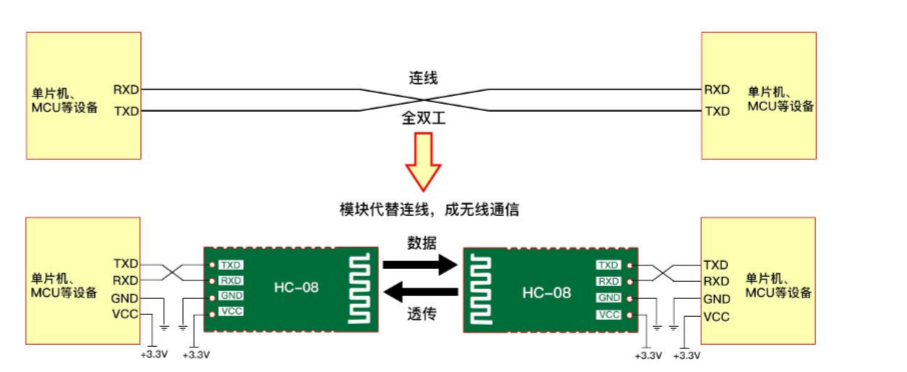### 2、测试模块是否正常工作，以及相关参数的设定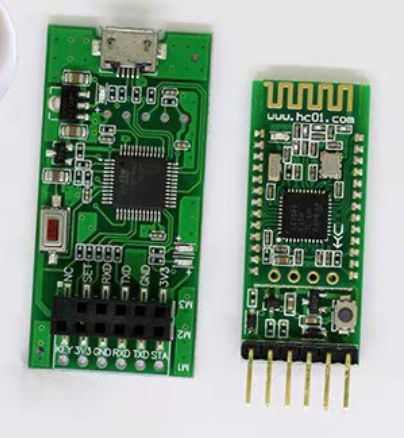### 3、蓝牙模块与单片机的连接，与手机的通信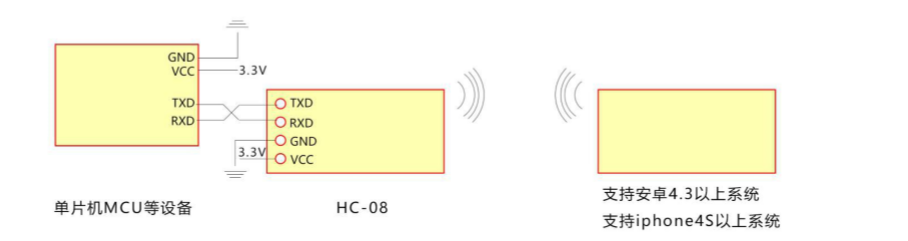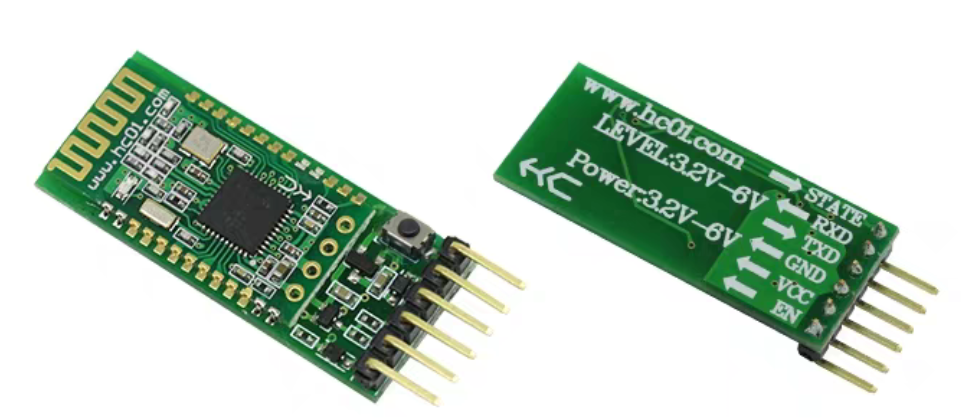##### 在这里需要确定你用的蓝牙模块的工作电压是多少，汇承HC-08有贴片装和带底板装两种，建议新手一定要买带底板的，如上图所示，可以省去很多麻烦。我们使用的51单片机供电电压是5v，而贴片装电压3.3v，不能直接与单片机连接，虽然本文介绍的这款单片机最小系统有3.3v的输出引脚，但是它串口TX RX依然是工作在5v电压下，而贴片装蓝牙模块的TX，RX需要工作在3.3v电压下，蓝牙模块的 RX 端需要串接一个 220Ω~1KΩ的电阻再接到单片机上，TX可以直接连接，对新手来说有点小麻烦，所以大家买的时候，直接买带底板的，支持3.2v-6v，也就是说带底板的可以直接与51单片机连接， 连接时只需要连4根线 Vcc连Vcc，GND连接GND，蓝牙模块TX接单片机 Rx （对于前文介绍的这款的单片机，RX为P30管脚，TX为P31管脚，如下图所示），蓝牙模块RX接单片机 Tx。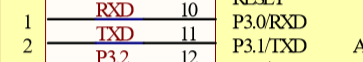## 二、蓝牙模块串口通信程序的编写以及蓝牙遥控的实现

### 1、与定时器相关函数的编写

##### 我们知道我们使用STC89C52单片机，只有两个定时器，在前文我们用定时器0，来实现电机的PWM输出，定时器1，来触发溢出中断，用于超声波模块障碍物的检测，而现在我们用蓝牙进行无线串口的通信，也需要用定时器，怎么办呢？ 定时器0肯定是不能动的，只能对定时器1进行复用，我们设定一个变量char Work_Mode=0; 进行工作模式的选择 为0时为手机或者电脑等上位机对小车进行蓝牙遥控 ，为1时小车自动避障模式，在一开始的时候，我们把它设为0，此时对定时器1初始化为蓝牙模块所需的工作模式，在我们需要转化成自动避障模式时，通过手机发送指令让Work_Mode变为1，此时我们把定时器1初始化为超声波避障所需的工作模式，由于此步是在while（1）循环中进行的，但是初始化只需要初始一次，不能一直初始化，所以需要再设定一个变量Work_Mode2，初始值设为0，当执行一次把定时器1初始化为超声波避障所需的工作模式的初始化后，就让该变量为1，这样就可以实现只初始化一次了，相关代码如下：
``````void Timer1Init()    //定时器1设定为为自动避障模式所需的初始化
{
TMOD=0X11;//选择为定时器1模式，工作方式1，仅用TR1打开启动。选择为定时器0模式，工作方式1，仅用TR1打开启动
TH1=0;
TL1=0;
ET1=1;//打开定时器1中断允许
EA=1;//打开总中断
TR1=1;//打开定时器
}

void Timer1Init2()   //定时器1设定为为蓝牙遥控模式所需的初始化
{
SCON=0X50;			//设置为工作方式1,8位数据，可变波特率
TMOD |=0X20;			//设置计数器工作方式2
PCON=0X00;			//波特率不加倍
TH1=0XFd;		    //计数器初始值设置，9600  @11.0592MHz
TL1=0XFd;
TR1=1;					//打开计数器
ES = 1;         //开串口中断
EA = 1;         //开总中断
}
``````
``````char Work_Mode=0;   //工作模式的选择  为0时，为手机或者电脑等上位机对小车进行蓝牙遥控 ，为1时小车自动避障模式
char Work_Mode2=0;

void main()
{
Timer0Init();
Timer1Init2();
Left_Speed_Ratio=5;   //设置左电机车速为最大车速的50%
Right_Speed_Ratio=5;	////设置右电机车速为最大车速的50%
while(1)
{
if(Work_Mode==1)
{
ES=0;   //关闭串口中断
if(Work_Mode2==0)
{
Timer1Init();
Work_Mode2=1;
}
if(Echo==1)
{
TH1=0;
TL1=0;
TR1=1;			    //开启计数
while(Echo);			//当RX为1计数并等待
TR1=0;				//关闭计数
Conut();			//计算
}
if(M_sensor==1)
{  run(); }
else
{
if(L_sensor==1)
{     left();       }

else if(R_sensor==1)
{    right() ;      }
else
{    back();          }
}

}
else

{

switch(receive_real_data)
{

case '1': run(); break;
case '2': left(); break;
case '3': right(); break;
case '4': back(); break;
case '5': Speed_add(); break;
case '6': Speed_reduce(); break;
case '7': stop(); break;
case '8': Work_Mode=1; break;
}

LED=1;
}
}
}

``````

### 2、对上面的主函数的解释（蓝牙遥控的实现思路）

##### 本篇文章的功能是在已经完成自动避障的基础上进行扩展的，也就是让小车可以具备自动避障和蓝牙遥控两种工作模式，所以当Work_Mode=1时的程序，也就是工作在自动避障模式的程序，在对定时器1完成设定为自动避障模式所需的初始化后，跟本系列第三篇文章介绍的是相同的，已详细介绍过了，在这就不介绍了，一开始的时候Work_Mode=0，工作在蓝牙遥控模式，我设定的功能如上面的主函数所示，当手机或者电脑或者其他上位机对单片机（通过蓝牙模块）发送1的时候，让小车前行。发送2的时候 让小车左转，发送3的时候让小车右转，发送4的时候，让小车后退，发送5的时候，让小车加速，发送6的时候让小车减速，发送7的时候，停车，发送8的时候，把Work_Mode置为1，使小车进入自动避障模式，此时蓝牙遥控失去作用，按下单片机上的复位键，程序将重新加载，重新进入蓝牙遥控模式。新增的加速减速函数如下：
``````void  Speed_add() //加速函数
{
if(Left_Speed_Ratio<10) //限幅
{
Left_Speed_Ratio++;
Right_Speed_Ratio++;
}
}

void  Speed_reduce() //减速函数
{
if(Left_Speed_Ratio>0)  //限幅
{
Left_Speed_Ratio--;
Right_Speed_Ratio--;
}
}
``````

### 3、串口中断函数的编写

##### 上面呢我们已经介绍了，通过发送一些设定好了的指令，实现蓝牙遥控，那么怎么把指令发送出去，以及单片机是如何实现接收的呢？，这就要通过串口中断来实现了，下面是官方给的一个串口中断的参考代码，是有问题的，对于HC-COM来说，是不能正常工作的
``````void Com_Int(void) interrupt 4
{

uchar receive_data;

EA = 0;

if(RI == 1) //当硬件接收到一个数据时，RI会置位
{
RI = 0;
receive_data = SBUF;//接收到的数据

if(receive_data == '1')
{
LED =0;//接收到1亮灯
}
else
{
LED =1; //其他情况灯灭
}

}
SBUF=receive_data;//将接收到的数据放入到发送寄存器
while(!TI);			 //等待发送数据完成
TI=0;						 //清除发送完成标志位
EA = 1;
}
``````
##### 按照上面的代码，当我们利用手机上的app HC-COM向单片机发送1的的时候，接收的数据receive_data=1，此时呢LED应该等于0，也就是LED会被点亮，但是实际上它只会闪一下，这就说明，我们通过HC-COM发送1的时候，单片机先接收到我们发的1，之后又接收到其他的信息，在上面的程序中，我们让单片机把接收到的信息又通过蓝牙模块发送给手机，利用HC-COM进行显示，如下图所示：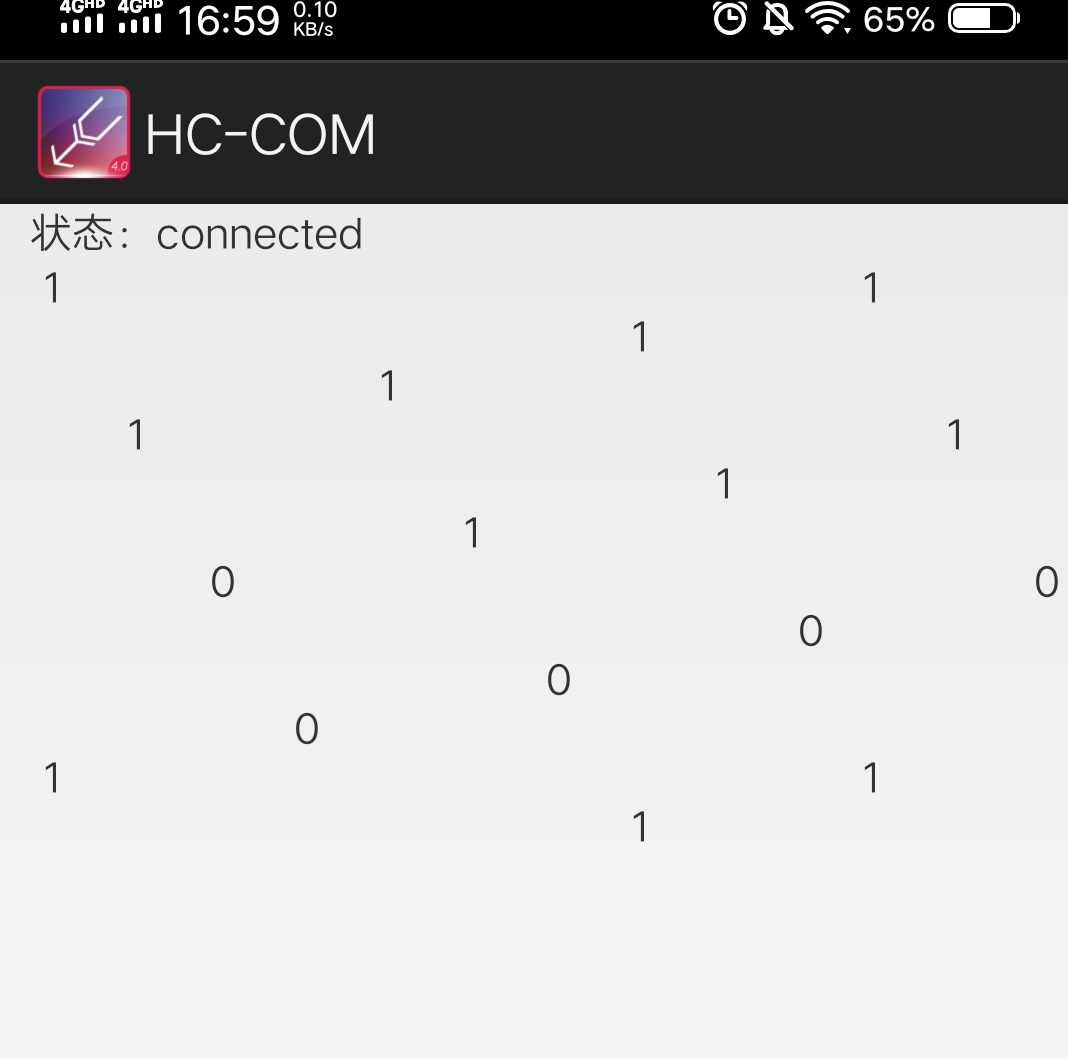##### 乍一看，我们发送1的时候，只返回了一个1 ，发送0的时候，只返回了一个0，那单片机接收的其他信息是从何而来的呢？，为啥手机没有显示返回的其他信息呢？对此我进行了大量的实验，最终发现这个app，它一次发送实际上是发送20位，什么意思呢？ 当我们输入一个1点击发送的时候，它实际上发送的是1000 0000 0000 0000 0000，也就是说当我们输入的数据的位数不足20位时它会自动补零，这就解释了为什么LED灯不会常亮，只会闪一下，因为在接收完我们发送的1后，它又接收了19个0，把这20个数据返回我们手机上的时候，它这个app把它补得这19个0又以空格的格式进行显示，而不是显示0（有点坑）。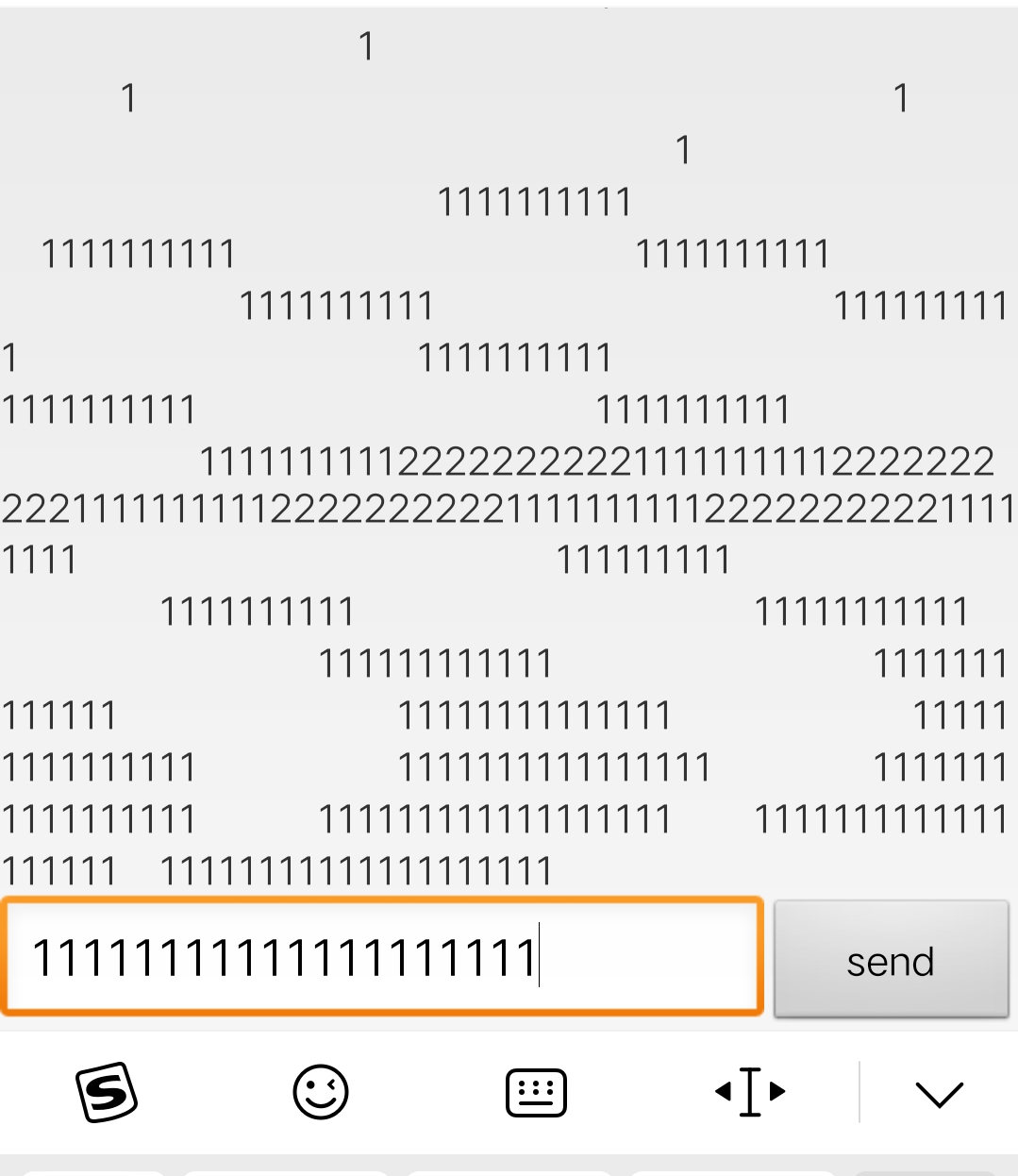##### 为了让LED常亮，我们需要输入20个1，如上图所示，也就是不给它补零的机会。这种方法呢用起来十分的不方便，而且本文我们要通过HC-COM发送指令，实现对小车的遥控，也就说需要我们快速的输入指令，这种方法是不行的，怎么办呢？，对大部分人了说不具备修改这个app的能力，那么只能修改单片机的接收和发送函数了，因此我把上面的串口中断函数进行了简单的修改，如下：
``````void Com_Int(void) interrupt 4
{
EA = 0;

if(RI == 1) //当硬件接收到一个数据时，RI会置位
{
LED=0;
RI = 0;
receive_data = SBUF;//接收到的数据
if(receive_data!=0)
receive_real_data=receive_data;

SBUF=receive_real_data;//将接收到的数据放入到发送寄存器
while(!TI);			 //等待发送数据完成
TI=0;						 //清除发送完成标志位

}
EA = 1;
}
``````

## 三、各文件完整的程序代码

### 1、main.c文件

``````#include <car.h>

extern unsigned char Left_Speed_Ratio;
extern unsigned char Right_Speed_Ratio;
unsigned int time=0;
unsigned int HC_SR04_time=0;
extern unsigned char pwm_val_left;
extern unsigned char pwm_val_right;
bit   flag =0;
extern char M_sensor;
char Work_Mode=0;   //工作模式的选择  为0时，为手机或者电脑等上位机对小车进行蓝牙遥控 ，为1时小车自动避障模式
char Work_Mode2=0;
unsigned char receive_data=0;
unsigned char receive_real_data=0;
void delay1s(void)
{
unsigned char a,b,c;
for(c=167;c>0;c--)
for(b=171;b>0;b--)
for(a=16;a>0;a--);
_nop_();
}
void delay1ms(void)
{
unsigned char a,b,c;
for(c=1;c>0;c--)
for(b=142;b>0;b--)
for(a=2;a>0;a--);
}

void Timer0Init()
{
TMOD|=0X01;//选择为定时器0模式，工作方式1，仅用TR0打开启动。

TH0=0XFC;	//给定时器赋初值，定时1ms
TL0=0X18;
ET0=1;//打开定时器0中断允许
EA=1;//打开总中断
TR0=1;//打开定时器
}

void Timer1Init()
{
TMOD=0X11;//选择为定时器1模式，工作方式1，仅用TR1打开启动。选择为定时器0模式，工作方式1，仅用TR1打开启动
TH1=0;
TL1=0;
ET1=1;//打开定时器1中断允许
EA=1;//打开总中断
TR1=1;//打开定时器
}

void Timer1Init2()
{
SCON=0X50;			//设置为工作方式1,8位数据，可变波特率
TMOD |=0X20;			//设置计数器工作方式2
PCON=0X00;			//波特率不加倍
TH1=0XFd;		    //计数器初始值设置，9600  @11.0592MHz
TL1=0XFd;
TR1=1;					//打开计数器
ES = 1;         //开串口中断
EA = 1;         //开总中断
}

void timer0()interrupt 1 using 2
{
TH0=0XFC;	//给定时器赋初值，定时1ms
TL0=0X18;
time++;
pwm_val_left++;
pwm_val_right++;
pwm_out_left_moto();
pwm_out_right_moto();

HC_SR04_time++;
if(HC_SR04_time>=500)   //500ms 启动一次超声波测距
{
HC_SR04_time=0;
StartModule();
}
}

void Timer1() interrupt 3
{
flag=1;    //若定时器1溢出则flag置1
}

void Com_Int(void) interrupt 4
{
EA = 0;

if(RI == 1) //当硬件接收到一个数据时，RI会置位
{
LED=0;
RI = 0;
receive_data = SBUF;//接收到的数据
if(receive_data!=0)
receive_real_data=receive_data;

SBUF=receive_real_data;//将接收到的数据放入到发送寄存器
while(!TI);			 //等待发送数据完成
TI=0;						 //清除发送完成标志位

}
EA = 1;
}

void main()
{
Timer0Init();
Timer1Init2();
Left_Speed_Ratio=5;   //设置左电机车速为最大车速的50%
Right_Speed_Ratio=5;	////设置右电机车速为最大车速的50%
while(1)
{
if(Work_Mode==1)
{
ES=0;   //关闭串口中断

if(Work_Mode2==0)
{

Timer1Init();
Work_Mode2=1;

}

if(Echo==1)
{
TH1=0;
TL1=0;
TR1=1;			    //开启计数
while(Echo);			//当RX为1计数并等待
TR1=0;				//关闭计数
Conut();			//计算
}

if(M_sensor==1)
{  run(); }
else
{
if(L_sensor==1)
{     left();       }

else if(R_sensor==1)
{    right() ;      }
else
{    back();          }

}

}
else

{

switch(receive_real_data)
{

case '1': run(); break;
case '2': left(); break;
case '3': right(); break;
case '4': back(); break;
case '5': Speed_add(); break;
case '6': Speed_reduce(); break;
case '7': stop(); break;
case '8': Work_Mode=1; break;
}

LED=1;
}
}
}

``````

### 2、motor_control.c文件

``````#include <car.h>

unsigned char pwm_val_left =0;
unsigned char push_val_left =0;
unsigned char pwm_val_right =0;
unsigned char push_val_right=0;
unsigned char Left_Speed_Ratio;
unsigned char Right_Speed_Ratio;

bit Left_moto_stop =1;
bit Right_moto_stop =1;

void Left_moto_go()  //左电机正转
{p34=0;p35=1;}
void Left_moto_back() //左电机反转
{p34=1;p35=0;}
void Left_moto_stp()  //左电机停转
{p34=1;p35=1;}
void Right_moto_go()  //右电机正转
{p36=0;p37=1;}
void Right_moto_back() //右电机反转
{p36=1;p37=0;}
void Right_moto_stp()  //右电机停转
{p36=1;p37=1;}

void pwm_out_left_moto(void)    //左电机PWM
{
if(Left_moto_stop)
{
if(pwm_val_left<=push_val_left)
Left_moto_pwm=1;
else
Left_moto_pwm=0;
if(pwm_val_left>=10)
pwm_val_left=0;
}
else
Left_moto_pwm=0;
}

void pwm_out_right_moto(void)    //右电机PWM
{
if(Right_moto_stop)
{
if(pwm_val_right<=push_val_right)
Right_moto_pwm=1;
else
Right_moto_pwm=0;
if(pwm_val_right>=10)
pwm_val_right=0;
}
else
Right_moto_pwm=0;
}

void run(void)     //小车前行
{
push_val_left =Left_Speed_Ratio;
push_val_right =Right_Speed_Ratio;
Left_moto_go();
Right_moto_go();
}

void back(void)   //小车后退
{
push_val_left =Left_Speed_Ratio;
push_val_right =Right_Speed_Ratio;
Left_moto_back();
Right_moto_back();
}

void left(void)   //小车左转
{
push_val_left =Left_Speed_Ratio;
push_val_right =Right_Speed_Ratio;
Right_moto_go();
Left_moto_back();
}

void right(void) //小车右转
{
push_val_left =Left_Speed_Ratio;
push_val_right =Right_Speed_Ratio;
Right_moto_back();
Left_moto_go();
}

void stop(void)  //小车停止
{
push_val_left =Left_Speed_Ratio;
push_val_right =Right_Speed_Ratio;
Left_moto_stp();
Right_moto_stp();
}

void rotate(void) //小车原地转圈
{
push_val_left =Left_Speed_Ratio;
push_val_right =Right_Speed_Ratio;
Left_moto_back();
Right_moto_go();
}

void  Speed_add() //加速函数
{
if(Left_Speed_Ratio<10)
{
Left_Speed_Ratio++;
Right_Speed_Ratio++;
}
}

void  Speed_reduce() //减速函数
{
if(Left_Speed_Ratio>0)
{
Left_Speed_Ratio--;
Right_Speed_Ratio--;
}
}

``````

### 3、HC_SR04.c文件

``````#include <car.h>

float  S=0;
extern bit  flag;
unsigned int  measure_time;
char M_sensor;
void  StartModule() 		         //启动超声波模块
{
Trig=1;
_nop_();
_nop_();
_nop_();
_nop_();
_nop_();
_nop_();
_nop_();
_nop_();
_nop_();
_nop_();
_nop_();
_nop_();
_nop_();
_nop_();
_nop_();
_nop_();
_nop_();
_nop_();
_nop_();
_nop_();
_nop_();
Trig=0;
}

void Conut(void)
{
measure_time=TH1*256+TL1;
TH1=0;
TL1=0;
S=(measure_time*1.87)/100;     //算出来是CM
if( flag==1||S>50||S<2)		    //超出测量
{
flag=0;
LED=1;
M_sensor=1;
}
else
{
LED=0;
M_sensor=0;
}
}

``````

### 4、car.h文件

``````#ifndef __car_H
#define __car_H

#include <reg52.h>
#include <intrins.h>

sbit Left_moto_pwm=P1^6 ;
sbit Right_moto_pwm=P1^7;
sbit p34=P3^4;
sbit p35=P3^5;
sbit p36=P3^6;
sbit p37=P3^7;
sbit Trig= P1^4; //产生脉冲引脚
sbit Echo= P1^5; //回波引脚
sbit LED=P0^0;
sbit L_sensor=P2^0;
sbit R_sensor=P2^1;
void Left_moto_go() ;
void Left_moto_back() ;
void Left_moto_stp() ;
void Right_moto_go();
void Right_moto_back();
void Right_moto_stp();
void delay(unsigned int k) ;
void delay1s(void) ;
void delay1ms(void);
void pwm_out_left_moto(void) ;
void pwm_out_right_moto(void);
void run(void);
void back(void);
void left(void);
void right(void);
void stop(void);
void rotate(void);
void  StartModule() ;
void Timer1Init();
void Timer0Init();
void Conut(void);
void Timer1Init2();
void  Speed_add() ;
void  Speed_reduce();

#endif
``````

# 手机蓝牙控制效果视频展示链接

## 附小车照片：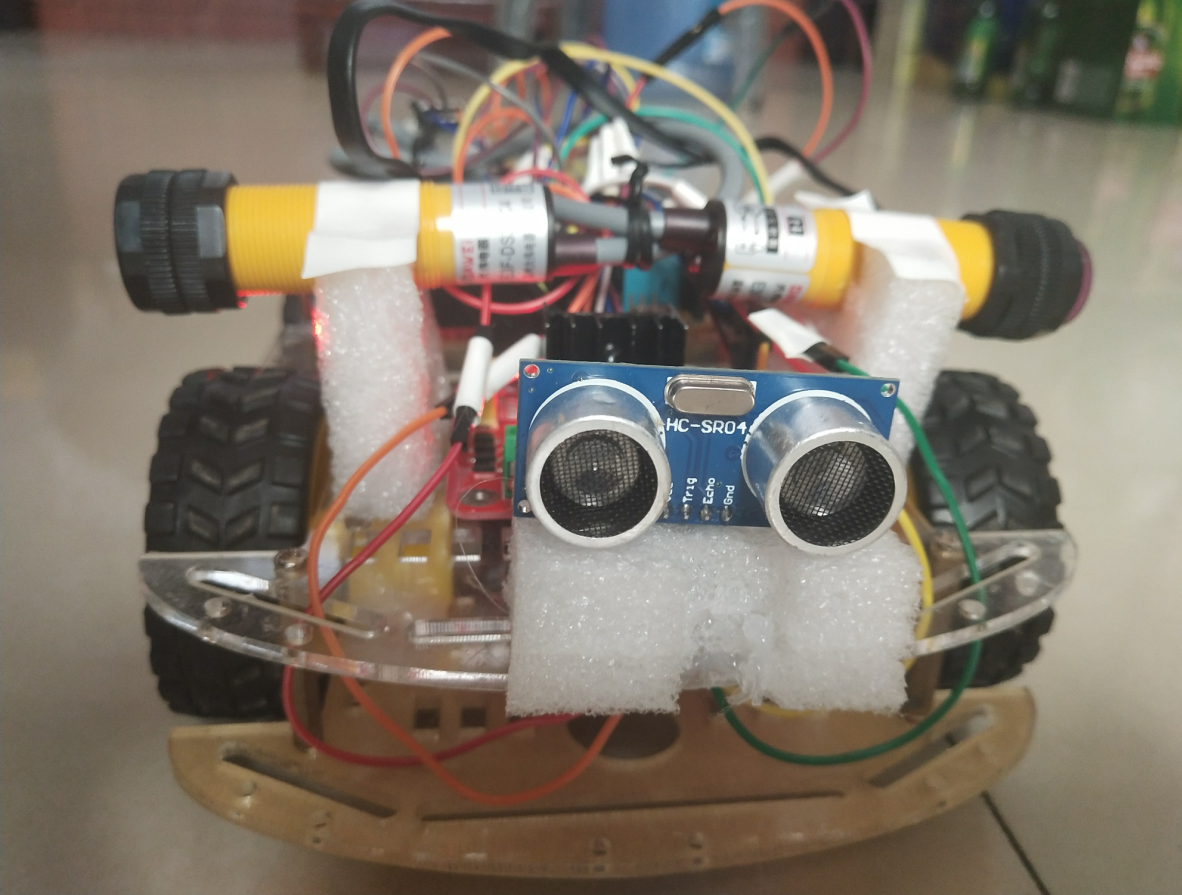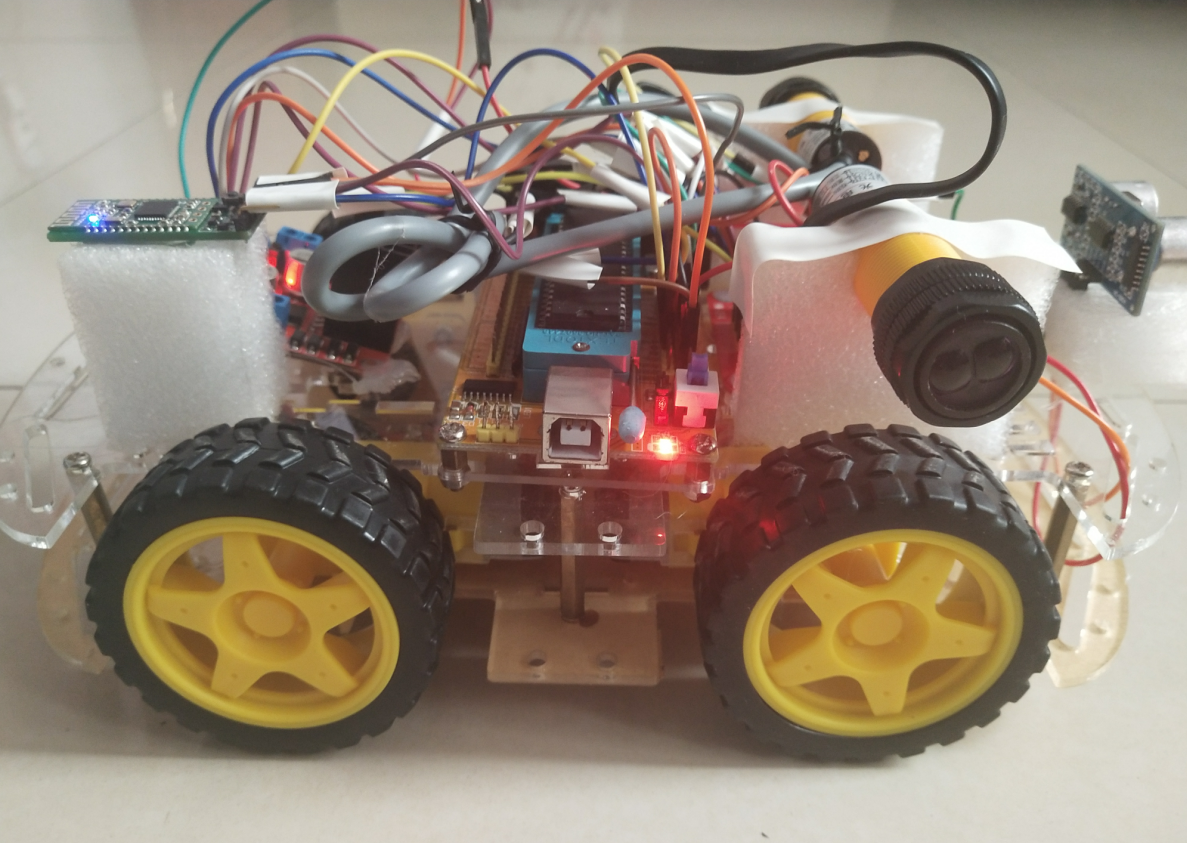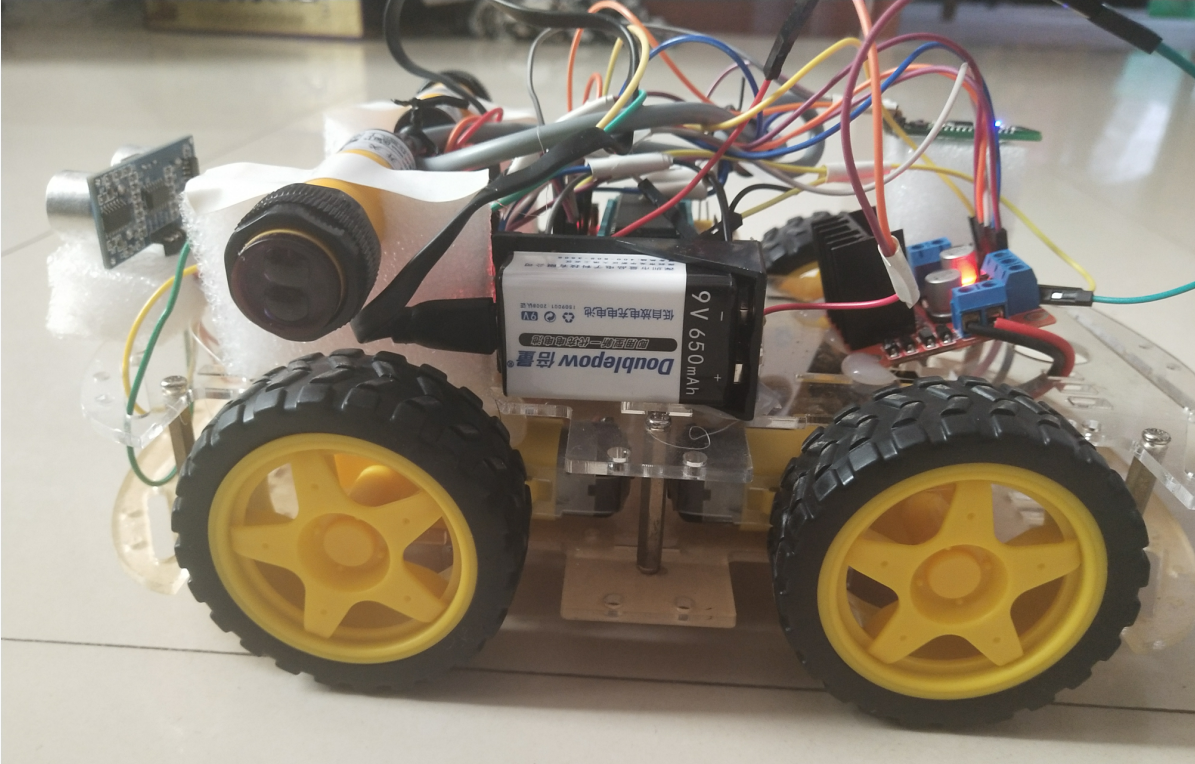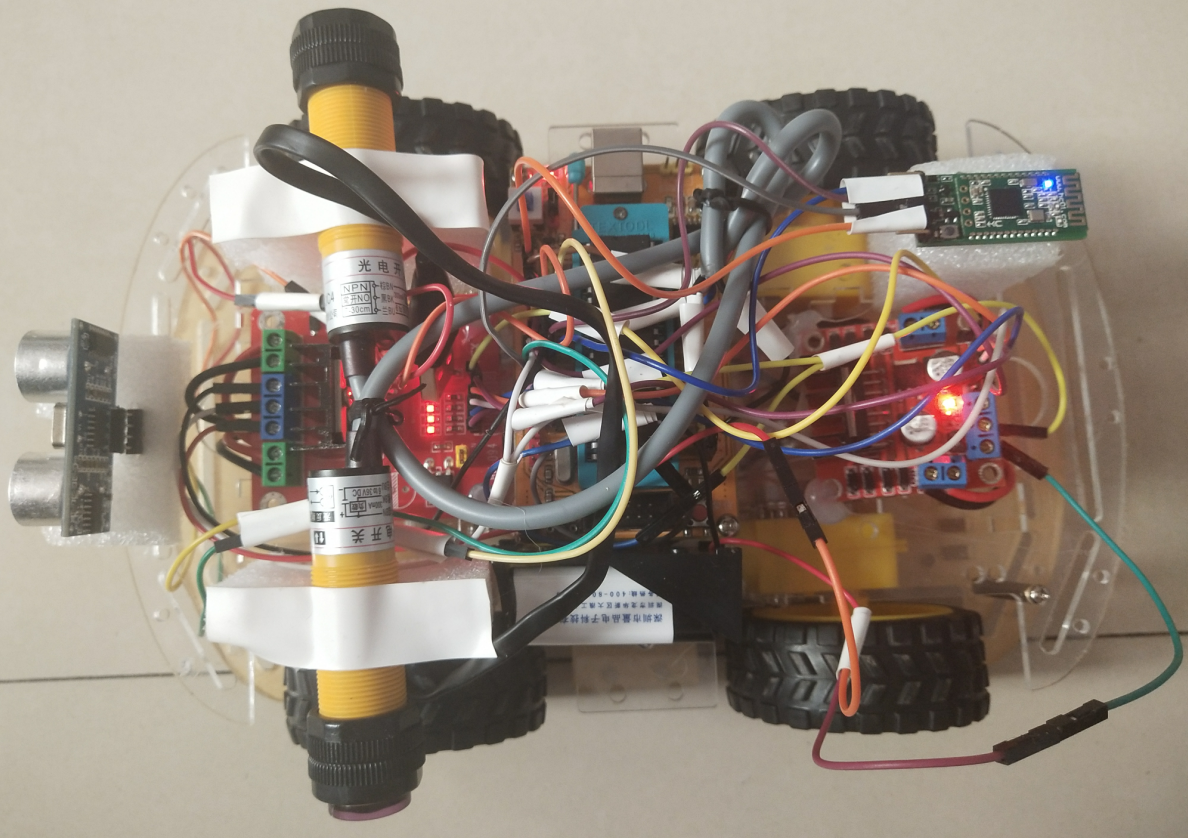本文介绍的内容的完整代码的keil文件和蓝牙HC—08模块的资料包（包括HC-COM这个app在内）我会放到附件里，还是那句话，我放的时候都是免费的，但是它会自己涨，需要的可以评论区留言，我直接发给你
欢迎大家积极交流，本文未经允许谢绝转载